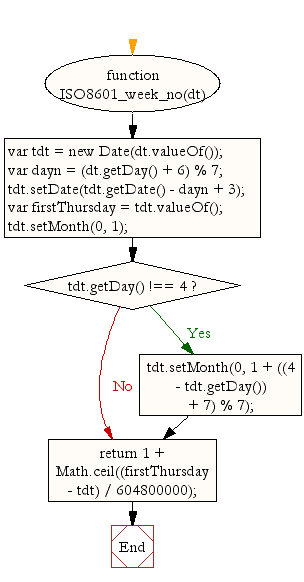# JavaScript: Get ISO-8601 week number of year, weeks starting on Monday

## JavaScript Datetime: Exercise-24 with Solution

Write a JavaScript function for obtaining ISO-8601 week numbers for weeks that begin on Monday.

Example : 42 (the 42nd week in the year)
Test Data :
dt = new Date(2015, 10, 1);
console.log(ISO8601_week_no(dt));
44

Sample Solution:-

HTML Code:

``````<!DOCTYPE html>
<html>
<meta charset="utf-8">
<title>JavaScript function to get ISO-8601 week number of year, weeks starting on Monday</title>
<body>

</body>
</html>
```
```

JavaScript Code:

``````function ISO8601_week_no(dt)
{
var tdt = new Date(dt.valueOf());
var dayn = (dt.getDay() + 6) % 7;
tdt.setDate(tdt.getDate() - dayn + 3);
var firstThursday = tdt.valueOf();
tdt.setMonth(0, 1);
if (tdt.getDay() !== 4)
{
tdt.setMonth(0, 1 + ((4 - tdt.getDay()) + 7) % 7);
}
return 1 + Math.ceil((firstThursday - tdt) / 604800000);
}

dt = new Date();
console.log(ISO8601_week_no(dt));

dt = new Date(2015, 10, 1);
console.log(ISO8601_week_no(dt));
```
```

Sample Output:

```25
44
```

Flowchart:Live Demo:

See the Pen JavaScript - Get ISO-8601 week number of year, weeks starting on Monday-date-ex-24 by w3resource (@w3resource) on CodePen.

Improve this sample solution and post your code through Disqus

What is the difficulty level of this exercise?

Test your Programming skills with w3resource's quiz.

﻿

## JavaScript: Tips of the Day

The unary operator

```let num = 10;

const increaseNumber = () => num++;
const increasePassedNumber = number => number++;

const num1 = increaseNumber();
const num2 = increasePassedNumber(num1);

console.log(num1);
console.log(num2);
```

The unary operator ++ first returns the value of the operand, then increments the value of the operand. The value of num1 is 10, since the increaseNumber function first returns the value of num, which is 10, and only increments the value of num afterwards.
num2 is 10, since we passed num1 to the increasePassedNumber. number is equal to 10(the value of num1. Again, the unary operator ++ first returns the value of the operand, then increments the value of the operand. The value of number is 10, so num2 is equal to 10.

Ref: https://bit.ly/323Y0P6

We are closing our Disqus commenting system for some maintenanace issues. You may write to us at reach[at]yahoo[dot]com or visit us at Facebook